# 1、无输入参数+无返回值

``````+ (void)animateWithDuration:(NSTimeInterval)duration animations:(void (^)(void))animations;
``````

``````int main(int argc, const char * argv[]) {
@autoreleasepool {
//block定义
void (^blockWithoutInputAndOutput) (void) = ^(void){
NSLog(@"欢迎访问 www.99ios.com!");
};
//block调用
blockWithoutInputAndOutput();
}
return 0;
}
``````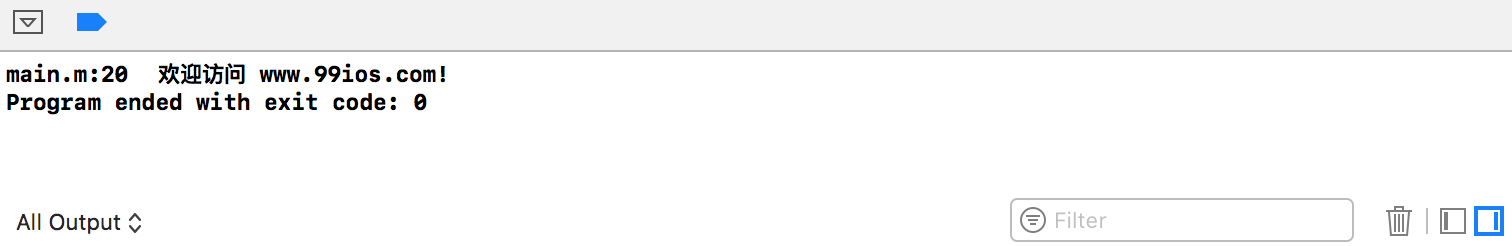# 2、有输入参数+无返回值

``````- (nullable NSURLSessionDataTask *)POST:(NSString *)URLString
parameters:(nullable id)parameters
``````

``````int main(int argc, const char * argv[]) {
@autoreleasepool {
//block的定义
void (^blockWithInput)(int) = ^(int inputNum) {
NSLog(@"blockWithInput被调用! 输入参数的值为 %d.",inputNum);
};
//block的调用
blockWithInput(99);
}
return 0;
}
``````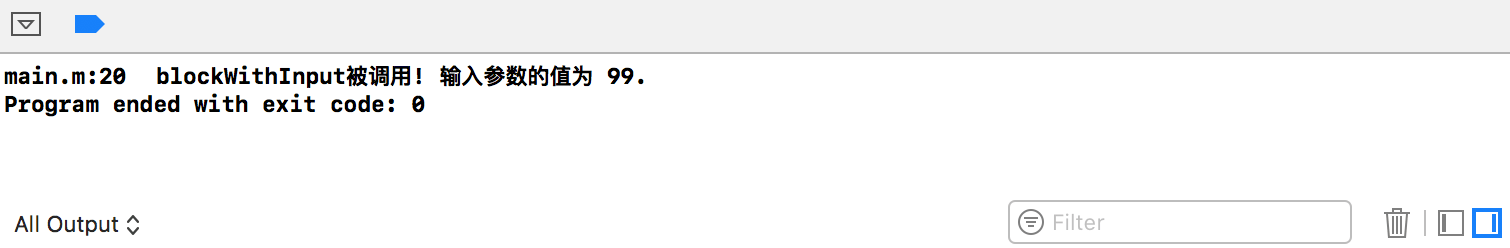# 3、有输入参数及返回值

Block中可以即有参数也有返回值，此时，需要在Block封装的代码中根据返回值的类型要求提供Block的返回值。例如，下方的示例代码中，blockWithOutputAndInput返回的是参数的平方(inputNum*inputNum)。

``````int main(int argc, const char * argv[]) {
@autoreleasepool {
//block的定义
int (^blockWithOutputAndInput)(int) = ^(int inputNum) {
NSLog(@"blockWithOutputAndInput被调用!");
return inputNum*inputNum;
};
//block的调用
int a = blockWithOutputAndInput(100);
NSLog(@"blockWithOutputAndInput的返回值是:%d",a);
}
return 0;
}
``````
• 运行结果：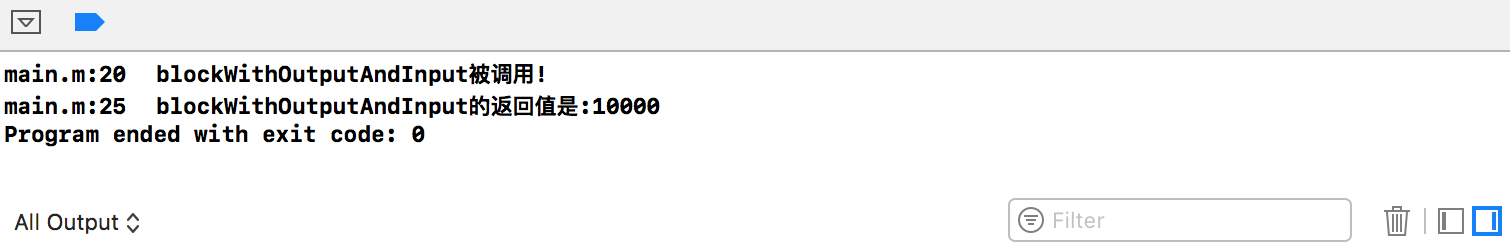# 4、有多个输入参数

• Block的定义与调用
``````int main(int argc, const char * argv[]) {
@autoreleasepool {
//block的定义
double (^multiplyTwoValues)(double, double) = ^(double number1, double number2) {
return number1 * number2;
};
//block的调用
double doubleNumber = multiplyTwoValues(5.0,5.6);
NSLog(@"multiplyTwoValues: %f",doubleNumber);
}
return 0;
}
``````
• 运行结果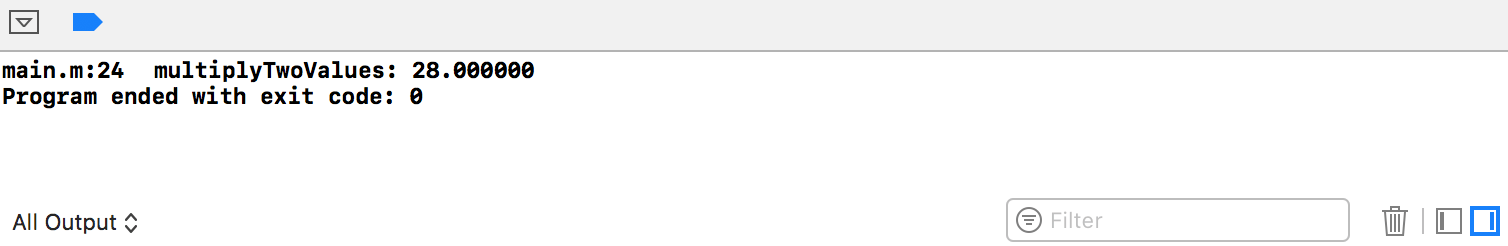# 5、无输入参数+有返回值

``````int main(int argc, const char * argv[]) {
@autoreleasepool {
//block的定义
int (^blockWithOutput) (void) = ^(void){
NSLog(@"blockWithOutput被调用!");
return 100;
};
//block的调用
int a = blockWithOutput();
NSLog(@"blockWithOutput的返回值:%d", a);
}
return 0;
}
``````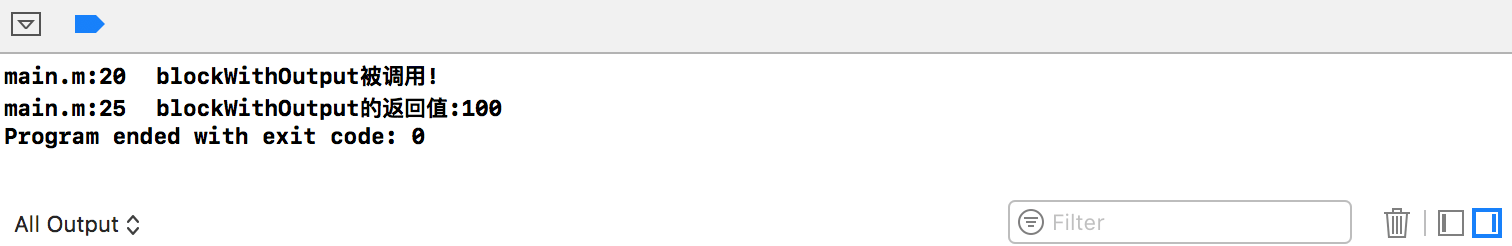# 示例代码

https://github.com/99ios/5.1.2